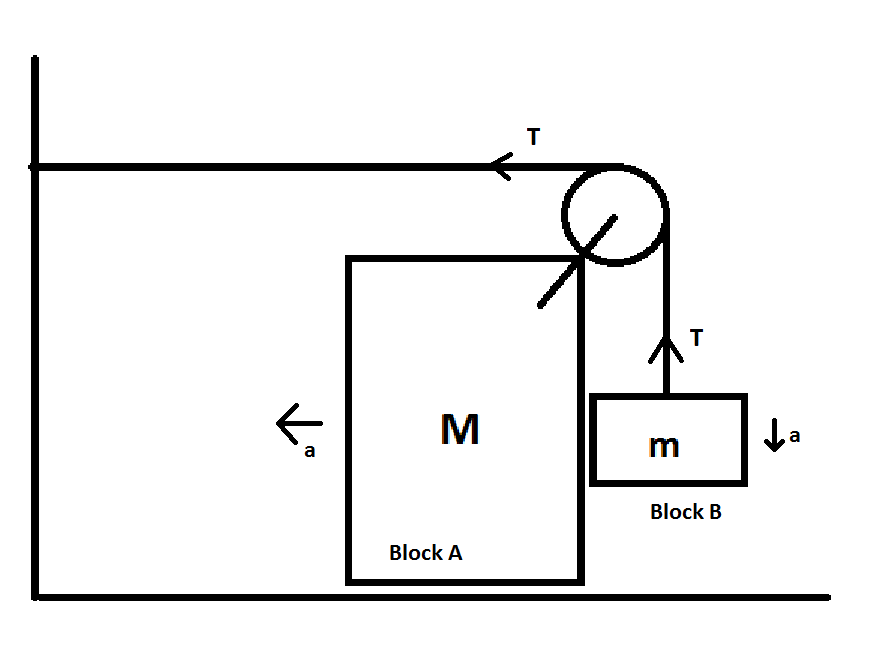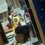# How is the block accelerating horizontally?Immediately after release, Block B would have some acceleration in the downward direction. Using constraint equations, we can easily prove that there would be an equal acceleration in Block A in the horizontal direction.

We have the following equations for the two blocks

Block A: T=Ma in the horizontal direction

Block B: mg-T=ma in the vertical direction

On solving, we have a=mg/(m+M)

But, wouldn't the block B also have horizontal acceleration? If Block A moves in the horizontal direction, wouldn't Block B also move with the same acceleration in this direction. But, if this is the case, what force is acting on the block in that direction? It cannot be a reaction force between the blocks as that would further push Block B away from A.

If Block B does not accelerate in the horizontal direction, how would the system behave after some time? (i.e. some instants after the release of the system) Would the distance between the blocks increase? I don't think that should happen. But, if that is not the case, what makes Block B move in the horizontal direction?Note by Deepani Agarwal
7 years, 2 months ago

This discussion board is a place to discuss our Daily Challenges and the math and science related to those challenges. Explanations are more than just a solution — they should explain the steps and thinking strategies that you used to obtain the solution. Comments should further the discussion of math and science.

When posting on Brilliant:

• Use the emojis to react to an explanation, whether you're congratulating a job well done , or just really confused .
• Ask specific questions about the challenge or the steps in somebody's explanation. Well-posed questions can add a lot to the discussion, but posting "I don't understand!" doesn't help anyone.
• Try to contribute something new to the discussion, whether it is an extension, generalization or other idea related to the challenge.

MarkdownAppears as
*italics* or _italics_ italics
**bold** or __bold__ bold
- bulleted- list
• bulleted
• list
1. numbered2. list
1. numbered
2. list
Note: you must add a full line of space before and after lists for them to show up correctly
paragraph 1paragraph 2

paragraph 1

paragraph 2

[example link](https://brilliant.org)example link
> This is a quote
This is a quote
    # I indented these lines
# 4 spaces, and now they show
# up as a code block.

print "hello world"
# I indented these lines
# 4 spaces, and now they show
# up as a code block.

print "hello world"
MathAppears as
Remember to wrap math in $$ ... $$ or $ ... $ to ensure proper formatting.
2 \times 3 $2 \times 3$
2^{34} $2^{34}$
a_{i-1} $a_{i-1}$
\frac{2}{3} $\frac{2}{3}$
\sqrt{2} $\sqrt{2}$
\sum_{i=1}^3 $\sum_{i=1}^3$
\sin \theta $\sin \theta$
\boxed{123} $\boxed{123}$

Sort by:

I got this.

- 7 years, 1 month ago